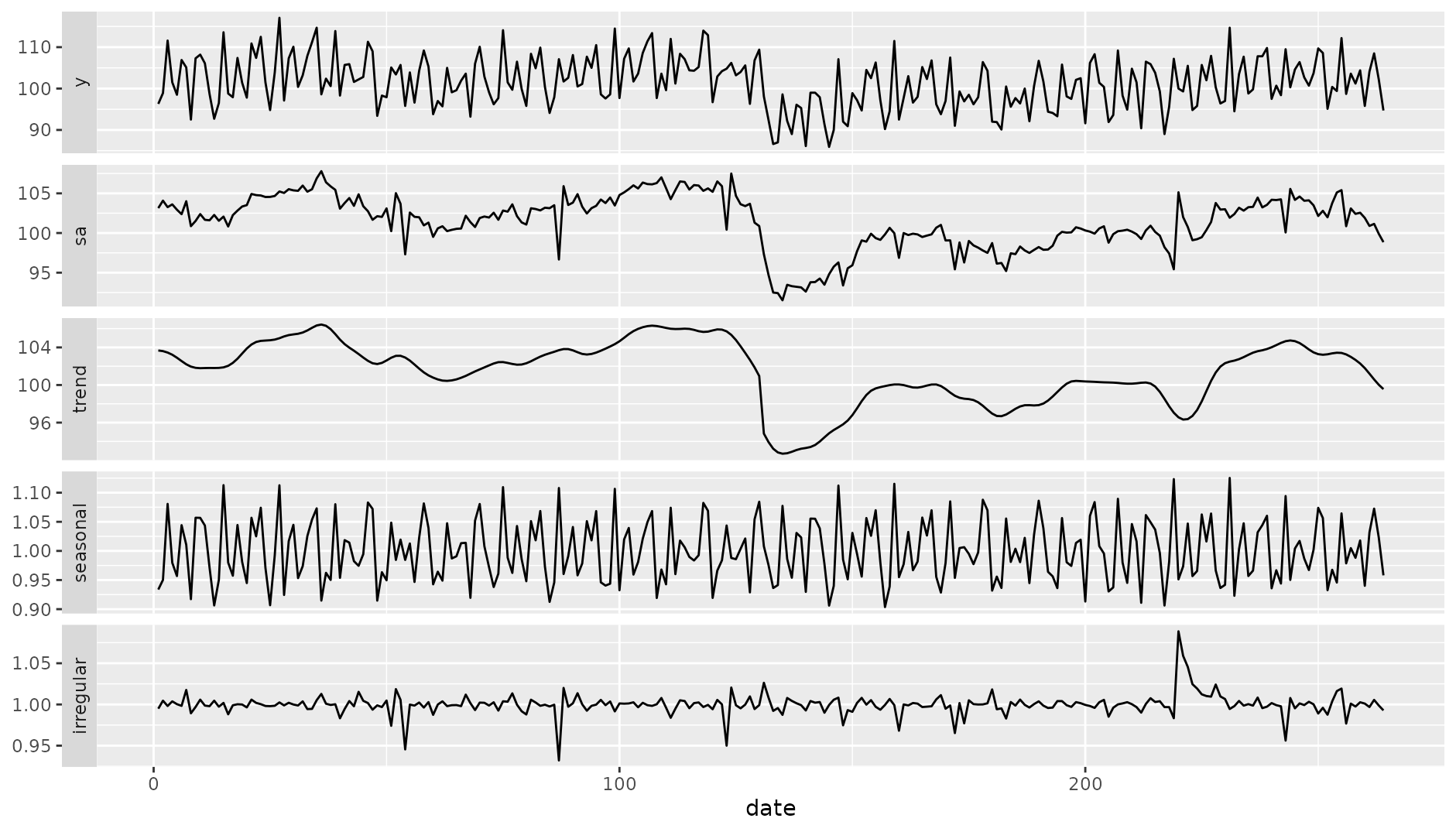ggdemetra is an extension of ggplot2 to add seasonal adjustment statistics to your plots. The seasonal adjustment process is done with RJDemetra that is an R interface to JDemetra+, the seasonal adjustment software officially recommended to the members of the European Statistical System (ESS) and the European System of Central Banks. RJDemetra implements the two leading seasonal adjustment methods TRAMO/SEATS+ and X-12ARIMA/X-13ARIMA-SEATS.

There are 4 main functionalities in ggdemetra depending of what you want to add in the graphic:

• geom_sa(): to add a time series compute during the seasonal adjustment (the trend, the seasonal adjusted time series, etc.).
• geom_outliers(): to add the outliers used in the pre-adjustment process of the seasonal adjustment.
• geom_arima(): to add the ARIMA model used in the pre-adjustment process of the seasonal adjustment.
• geom_diagnostics(): to add a table containing some diagnostics on the seasonal adjustment process.

Note ts objects cannot be directly used in ggplot2. To convert ts or mts object to data.frame, you can use the ts2df() function. For example, the data ipi_c_eu_df used in this package is obtained by applying the ts2df()function to the ipi_c_eu data available in RJDemetra:

ipi_c_eu_df <- ts2df(ipi_c_eu)

All the functions have some common parameters and especially those to defined the seasonal adjustment method:

• method is the method used for the seasonal adjustment: X-13ARIMA (method = "x13", the default) or TRAMO-SEATS (method = "tramoseats").
• spec is the seasonal adjustment specification. It can be the name of pre-defined specification (see ?RJDemetra::x13 or ?RJDemetra::tramoseats) or a user-defined specification created by RJDemetra (by RJDemetra::x13_spec or RJDemetra::tramoseats_spec).
• frequency is the frequency of the input time series. By default, the frequency is computed and a message is printed with the one chosen (use message = FALSE to suppress this message).

In the following examples, the data used is the French industrial production index. By default, the seasonal adjustment will then be processed with X-13ARIMA with a pre-defined specification "RSA5c (automatic log detection, automatic ARIMA and outliers detection and trading day and easter adjustment). However, in the industrial production the working day effect has more economic sense than the trading day effect and a gradual effect for easter does not make economic sense for the aggregated series. The specification that should be used with X-13ARIMA is spec = RJDemetra::x13_spec("RSA3", tradingdays.option = "WorkingDays"). If no new data or seasonal adjustment specification is specified (method or specification), these parameters is inherited from the previous defined: therefore you only need to specify this parameter once.

library(ggplot2)
library(ggdemetra)
p_ipi_fr <- ggplot(data = ipi_c_eu_df, mapping = aes(x = date, y = FR)) +
geom_line() +
labs(title = "Seasonal adjustment of the French industrial production index",
x = NULL, y = NULL)
p_ipi_fr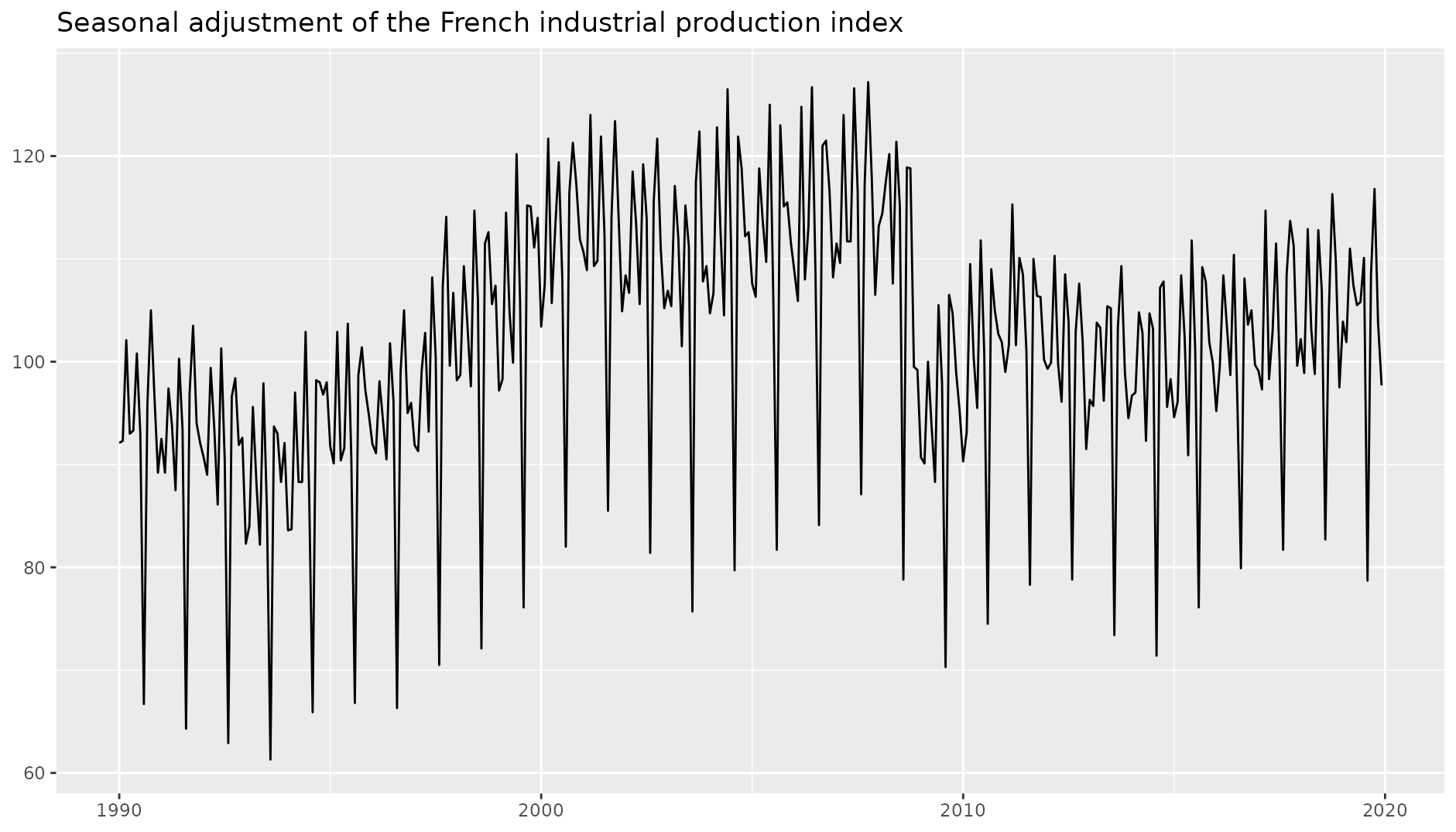By default geom_sa() adds the seasonal adjusted time series:

spec <- RJDemetra::x13_spec("RSA3", tradingdays.option = "WorkingDays")
p_ipi_fr +
geom_sa(color = "#155692",
spec = spec)
#> Frequency used: 12To add other components of the seasonal adjustment, use the component parameter of geom_sa() (see ?RJDemetra::user_defined_variables() for the availables parameters). For example, to add the forecasts of the input data and of the seasonal adjusted series:

p_sa <- p_ipi_fr +
geom_sa(component = "y_f", linetype = 2, message = FALSE,
spec = spec) +
geom_sa(component = "sa", color = "#155692") +
geom_sa(component = "sa_f", color = "#155692", linetype = 2)
p_sa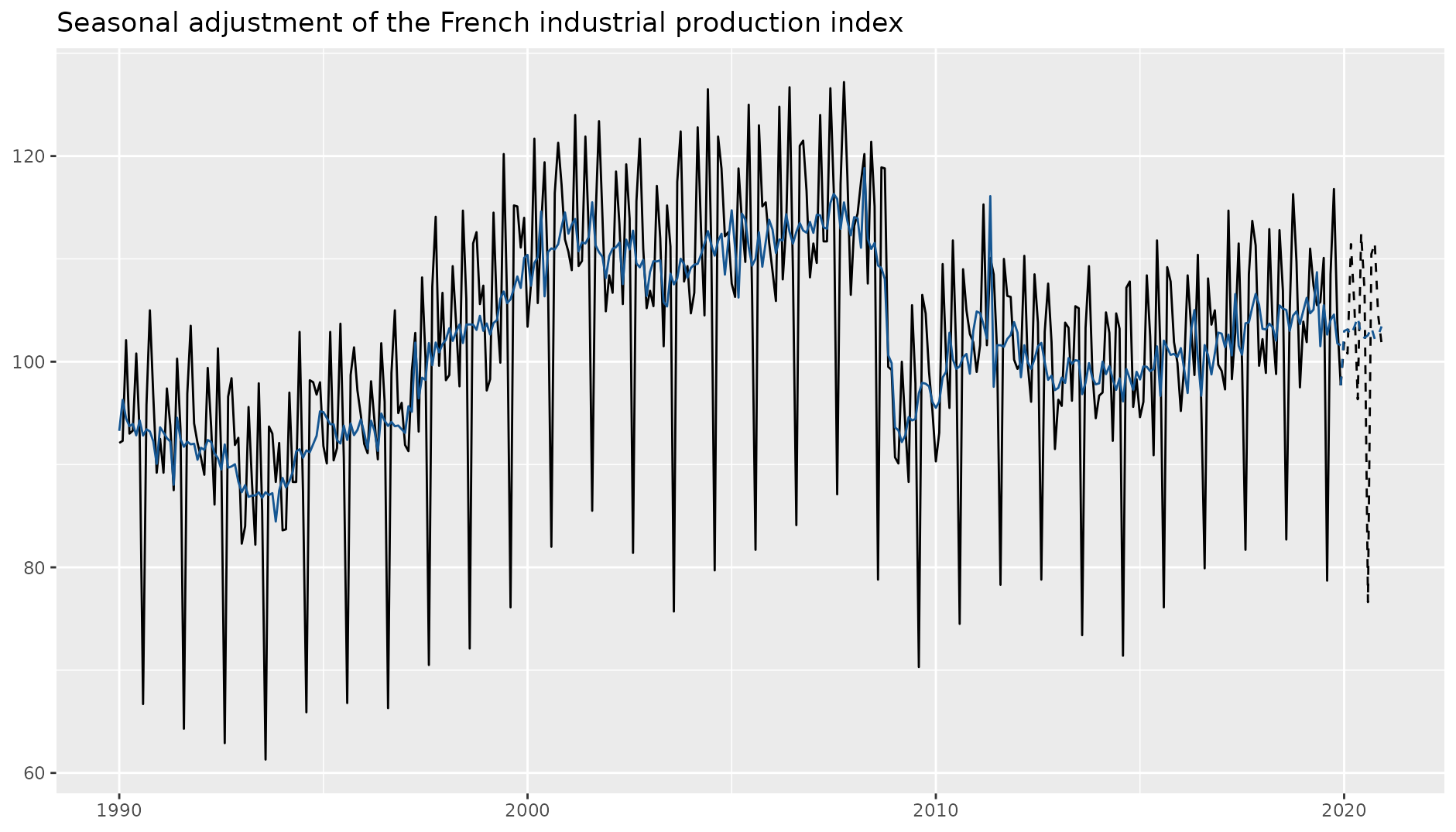## Add the outliers to the plot

There are four differents geometrics to add to the plot the names of the outliers used in the pre-adjustment process:

• geom = "text" (the default) adds directly the names of the outliers and geom = "label" draws a rectangle behind the names, making them easier to read.
• geom = "text_repel" and geom = "label_repel" do the same but text labels repel away from each other and away from the data points (see ?ggrepel::geom_label_repel).

In our example, there are 3 outliers:

p_sa + geom_outlier(geom = "label")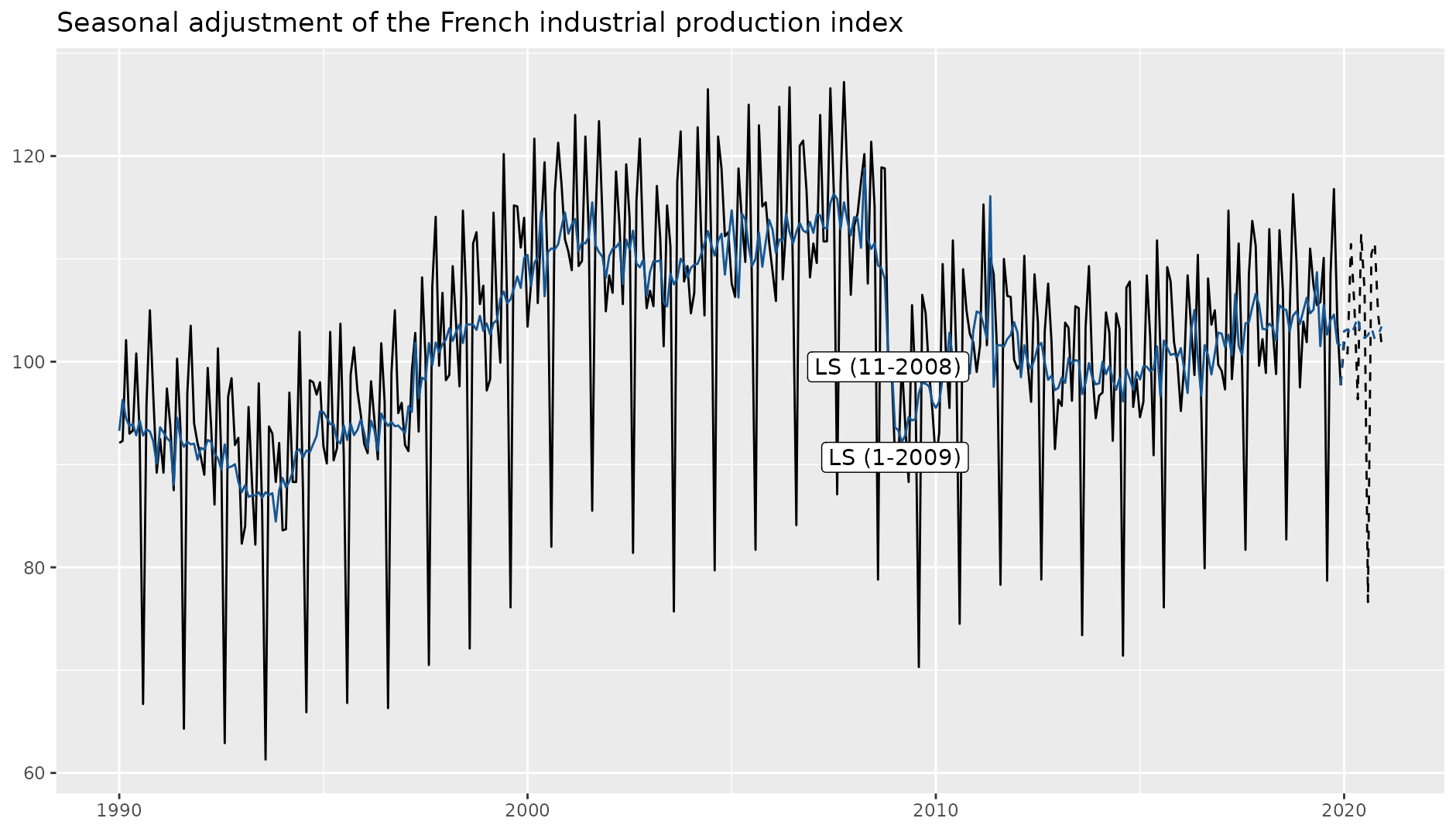They can be plotted in more readable way using the parameters of ggrepel::geom_label_repel:

p_sa +
geom_outlier(geom = "label_repel",
ylim = c(NA, 65),
arrow = arrow(length = unit(0.03, "npc"),
type = "closed", ends = "last"))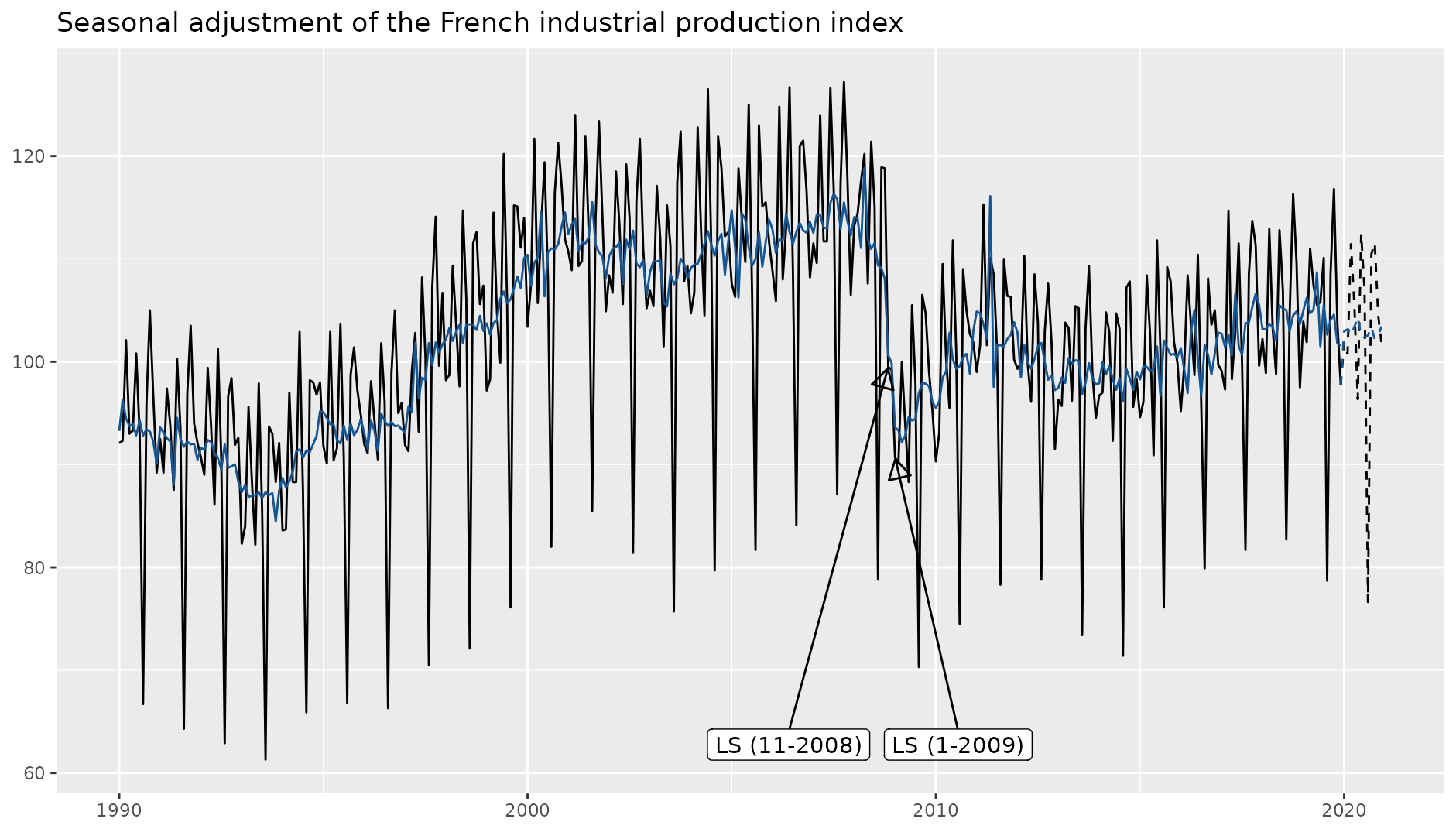Use the parameters first_date and last_date to only have the outliers in a precise time interval. For example, to only plot the outliers from 2009 use first_date = 2009:

p_sa +
geom_outlier(geom = "label_repel",
first_date = 2009,
ylim = c(NA, 65),
arrow = arrow(length = unit(0.03, "npc"),
type = "closed", ends = "last"))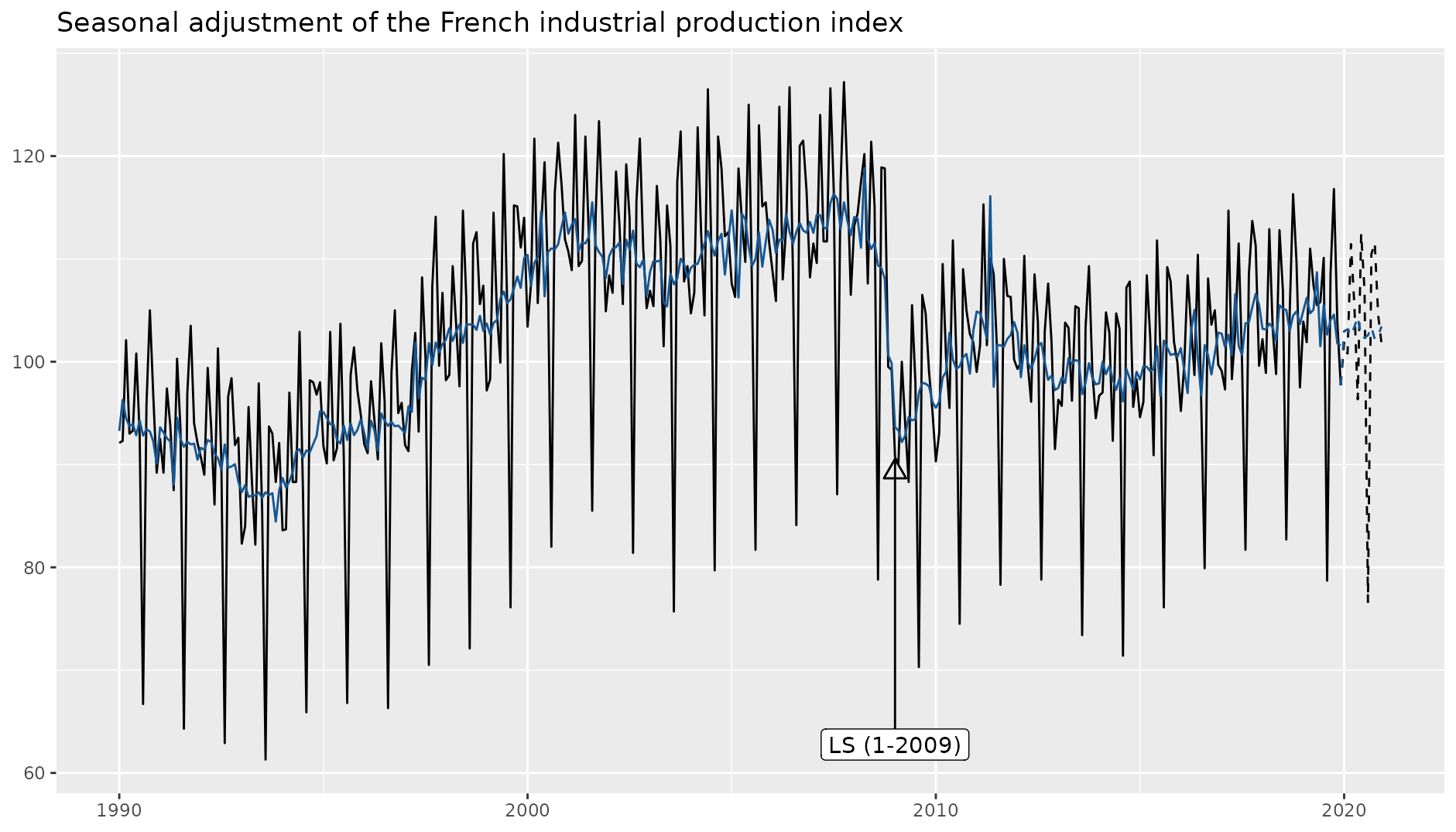The ARIMA model used pre-adjustment process can be added to the plot with geom_arima(). The parameter geom = "label" draws a rectangle behind the ARIMA model, making it easier to read:

p_sa +
geom_arima(geom = "label",
x_arima = -Inf, y_arima = -Inf,
vjust = -1, hjust = -0.1)## Add a table with some diagnostics

A table with some diagnostics on the seasonal adjustment process can be added with geom_diagnostics(). The desired diagnostics have to be added to the diagnostics parameter (see ?RJDemetra::user_defined_variables() for the availables diagnostics). For example, to add the result of the X-11 combined test and the p-values of the residual seasonality qs and f tests:

diagnostics <- c("diagnostics.combined.all.summary", "diagnostics.qs", "diagnostics.ftest")
p_sa +
geom_diagnostics(diagnostics = diagnostics,
ymin = 58, ymax = 72, xmin = 2010,
table_theme = gridExtra::ttheme_default(base_size = 8))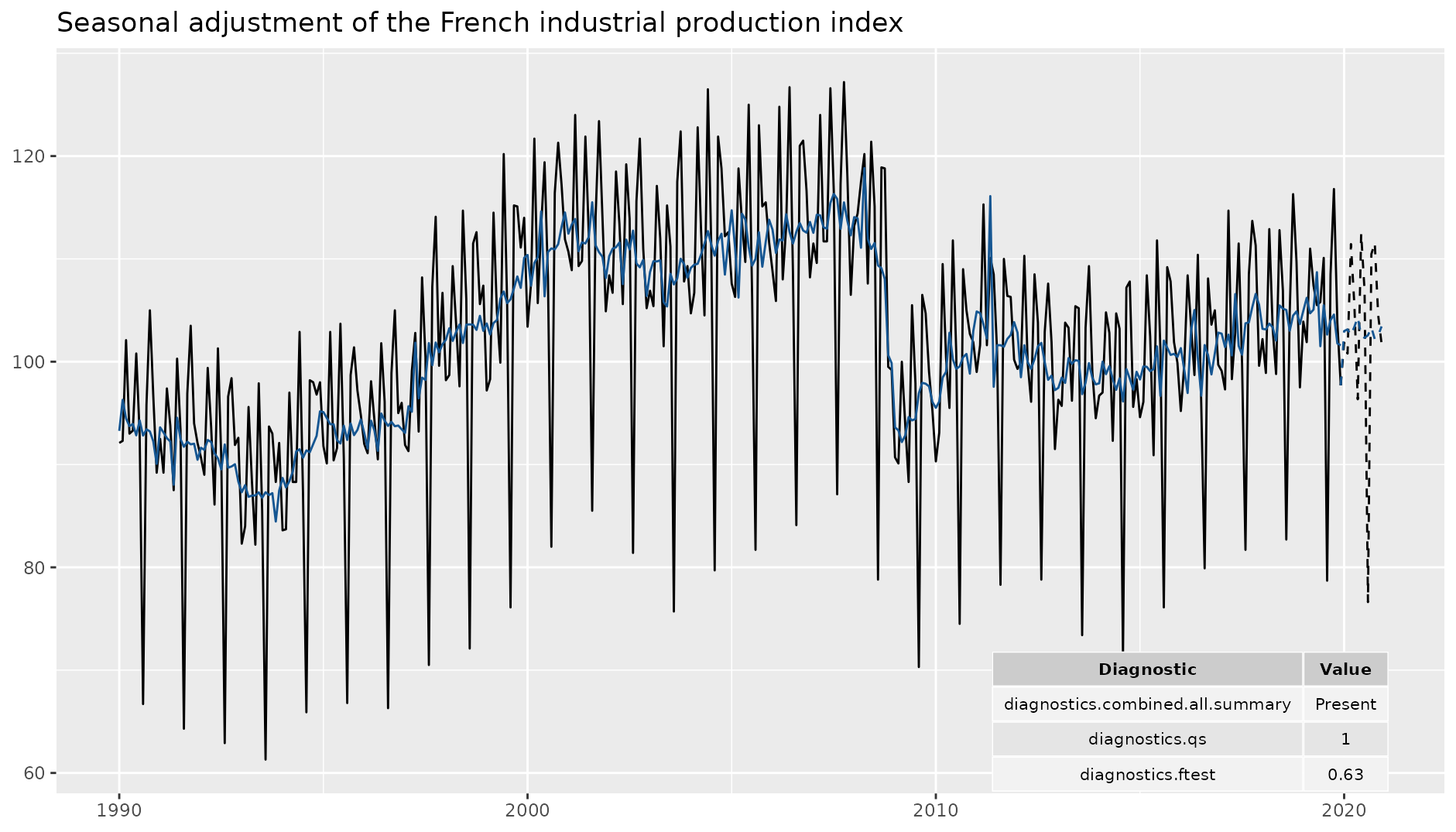To customize the names of the diagnostics in the plot, pass a named vector to the diagnostics parameter:

diagnostics <- c(Combined test = "diagnostics.combined.all.summary",
Residual qs-test (p-value) = "diagnostics.qs",
Residual f-test (p-value) = "diagnostics.ftest")
p_sa +
geom_diagnostics(diagnostics = diagnostics,
ymin = 58, ymax = 72, xmin = 2010,
table_theme = gridExtra::ttheme_default(base_size = 8))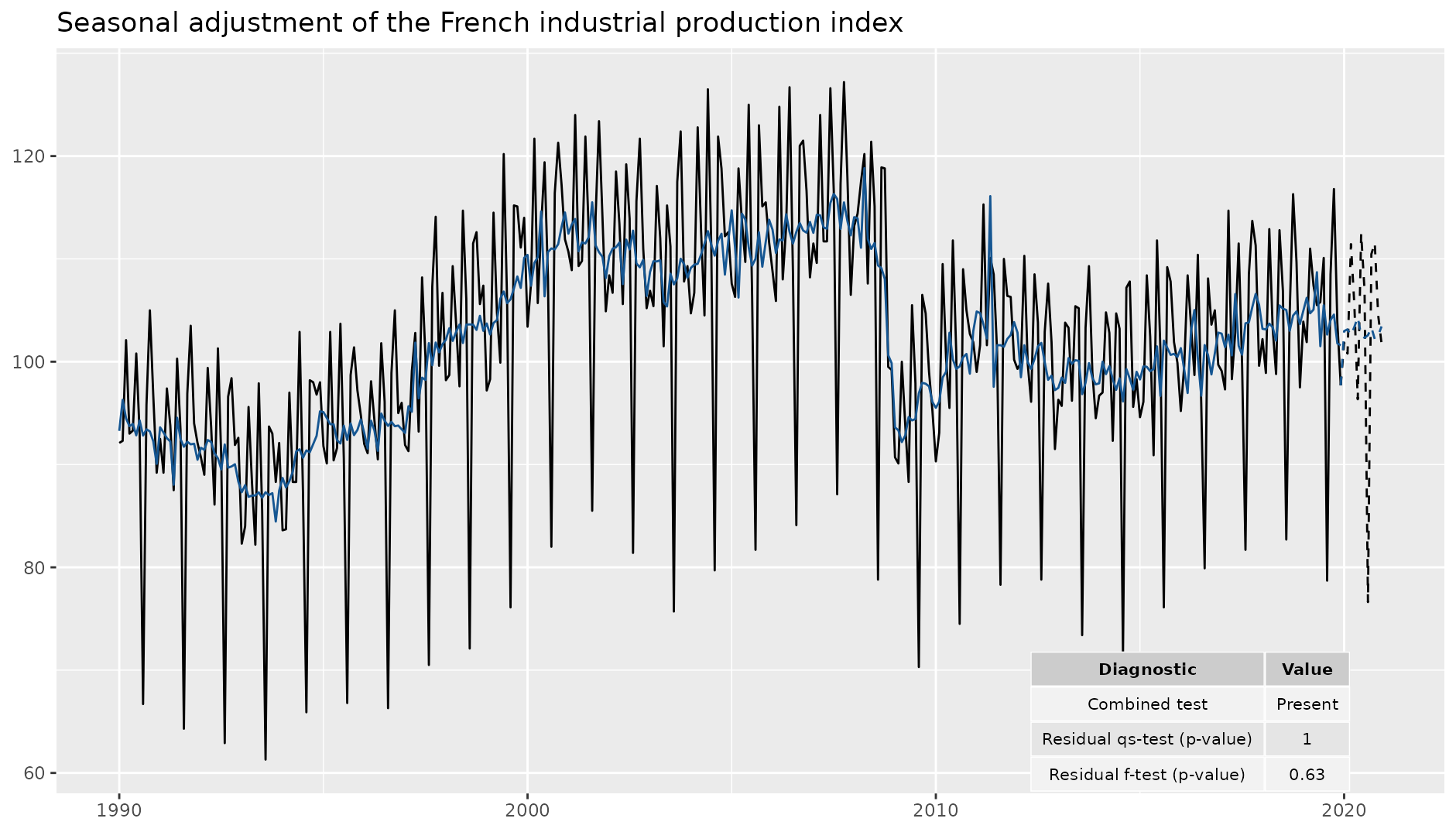To add the table below the plot, you can for example use gridExtra::grid.arrange():

p_diag <- ggplot(data = ipi_c_eu_df, mapping = aes(x = date, y = FR))  +
geom_diagnostics(diagnostics = diagnostics,
spec = spec, frequency = 12,
table_theme = gridExtra::ttheme_default(base_size = 8)) +
theme_void()

gridExtra::grid.arrange(p_sa, p_diag,
nrow = 2, heights  = c(4, 1.5))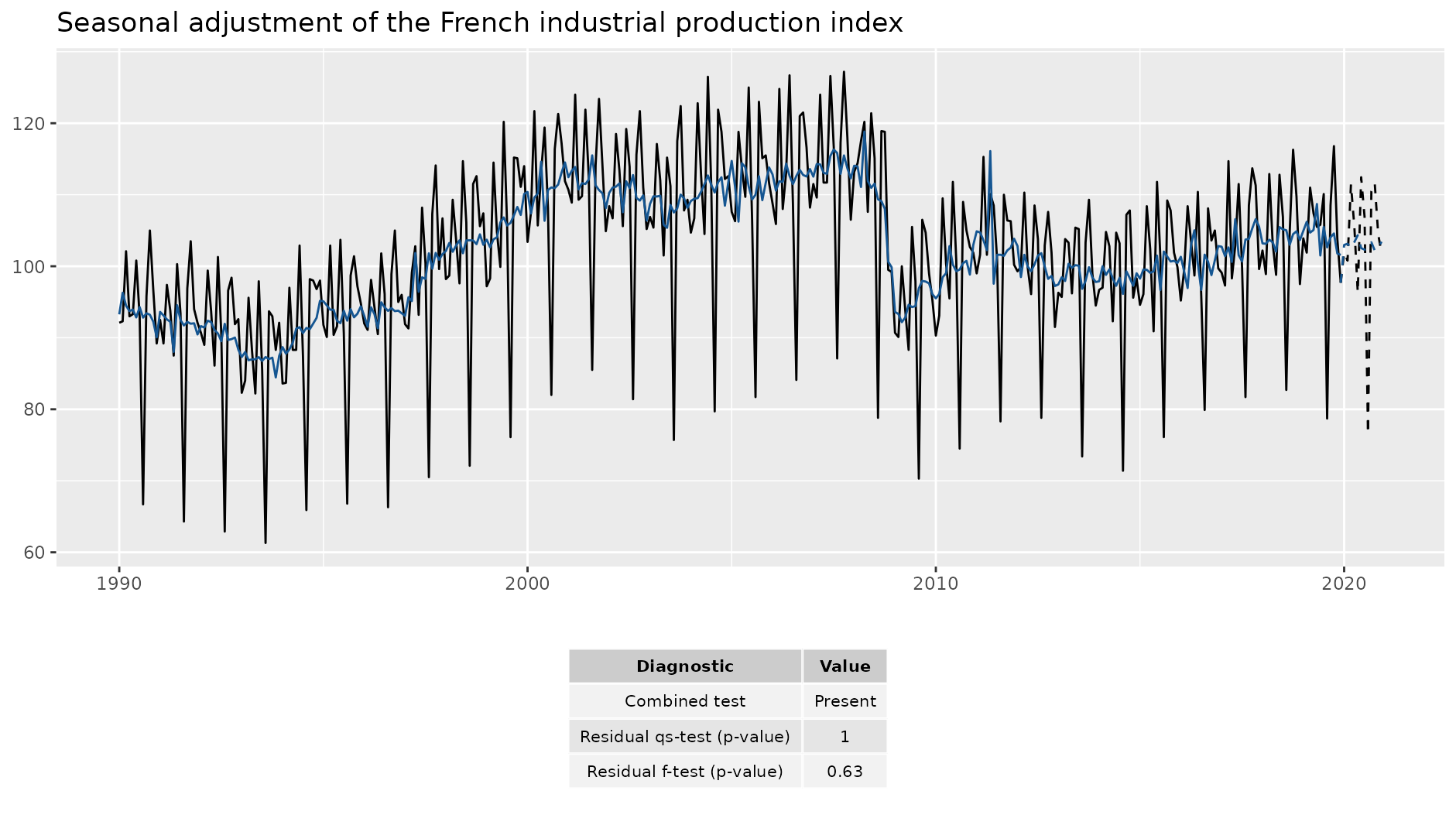## Use existing model

ggdemetra offers several function that can be used to manipulate existing models.

mod <- RJDemetra::x13(ipi_c_eu[,"UK"], spec)

The previous plots can be initialized with the init_ggplot() function:

init_ggplot(mod) +
geom_line(color =  "#F0B400") +
geom_sa(color =  "#155692") +
geom_arima(geom = "label",
x_arima = -Inf, y_arima = -Inf,
vjust = -1, hjust = -0.1)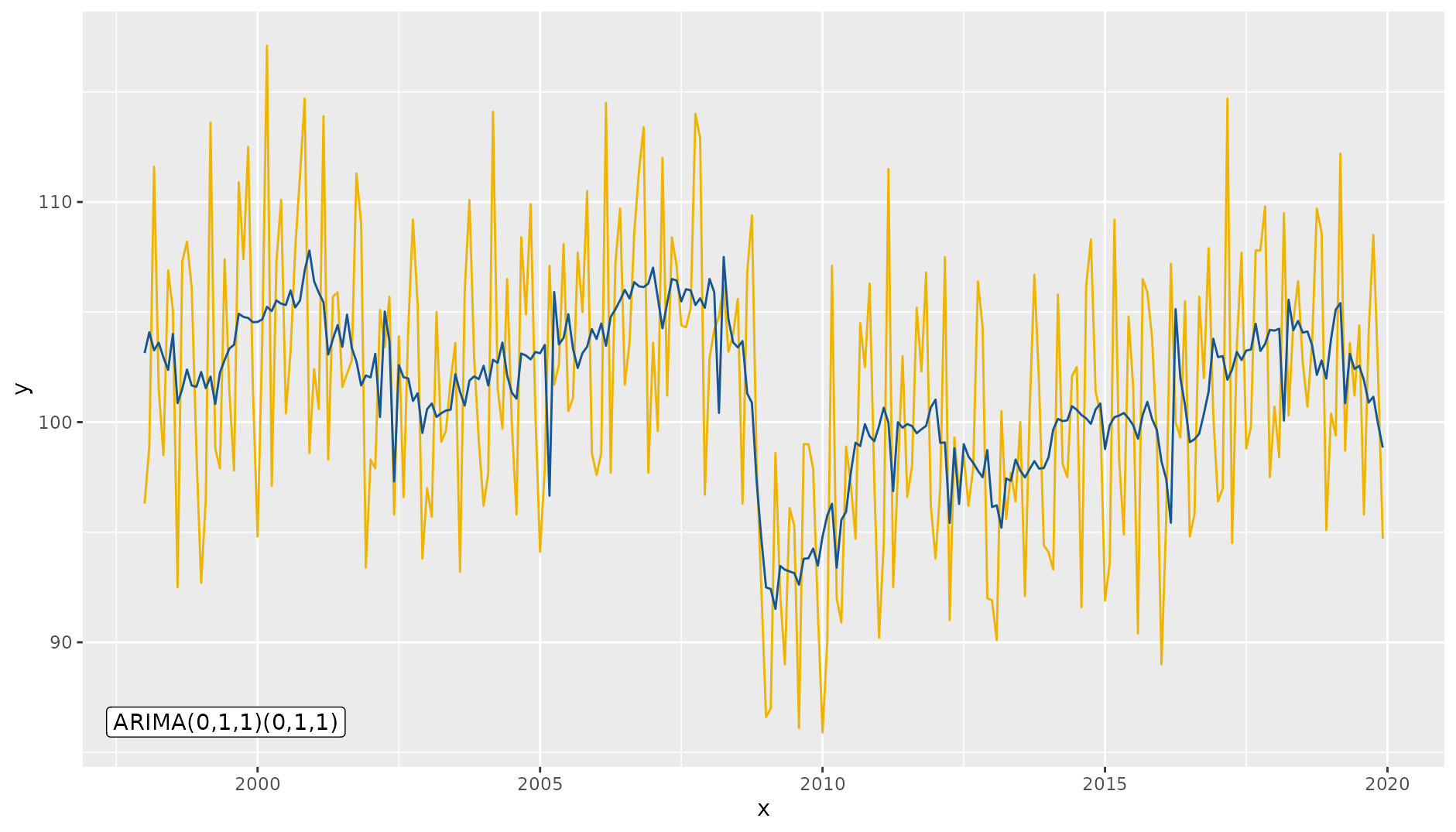The different components of seasonal adjustment models can be extracted through calendar(), calendaradj(), irregular(), trendcycle(), seasonal(), seasonaladj(), trendcycle() and raw().

data <- ts.union(raw(mod), raw(mod, forecast = TRUE),
trendcycle(mod), trendcycle(mod, forecast = TRUE),
colnames(data) <- c("y", "y_f",
"t", "t_f",
"sa", "sa_f")
ggplot(data = ts2df(data), mapping = aes(x = date)) +
geom_line(mapping = aes(y = y), color =  "#F0B400", na.rm = TRUE) +
geom_line(mapping = aes(y = y_f), color =  "#F0B400", na.rm = TRUE, linetype = 2) +
geom_line(mapping = aes(y = t), color =  "#1E6C0B", na.rm = TRUE) +
geom_line(mapping = aes(y = t_f), color =  "#1E6C0B", na.rm = TRUE, linetype = 2) +
geom_line(mapping = aes(y = sa), color =  "#155692", na.rm = TRUE) +
geom_line(mapping = aes(y = sa_f), color =  "#155692", na.rm = TRUE, linetype = 2) +
theme_bw()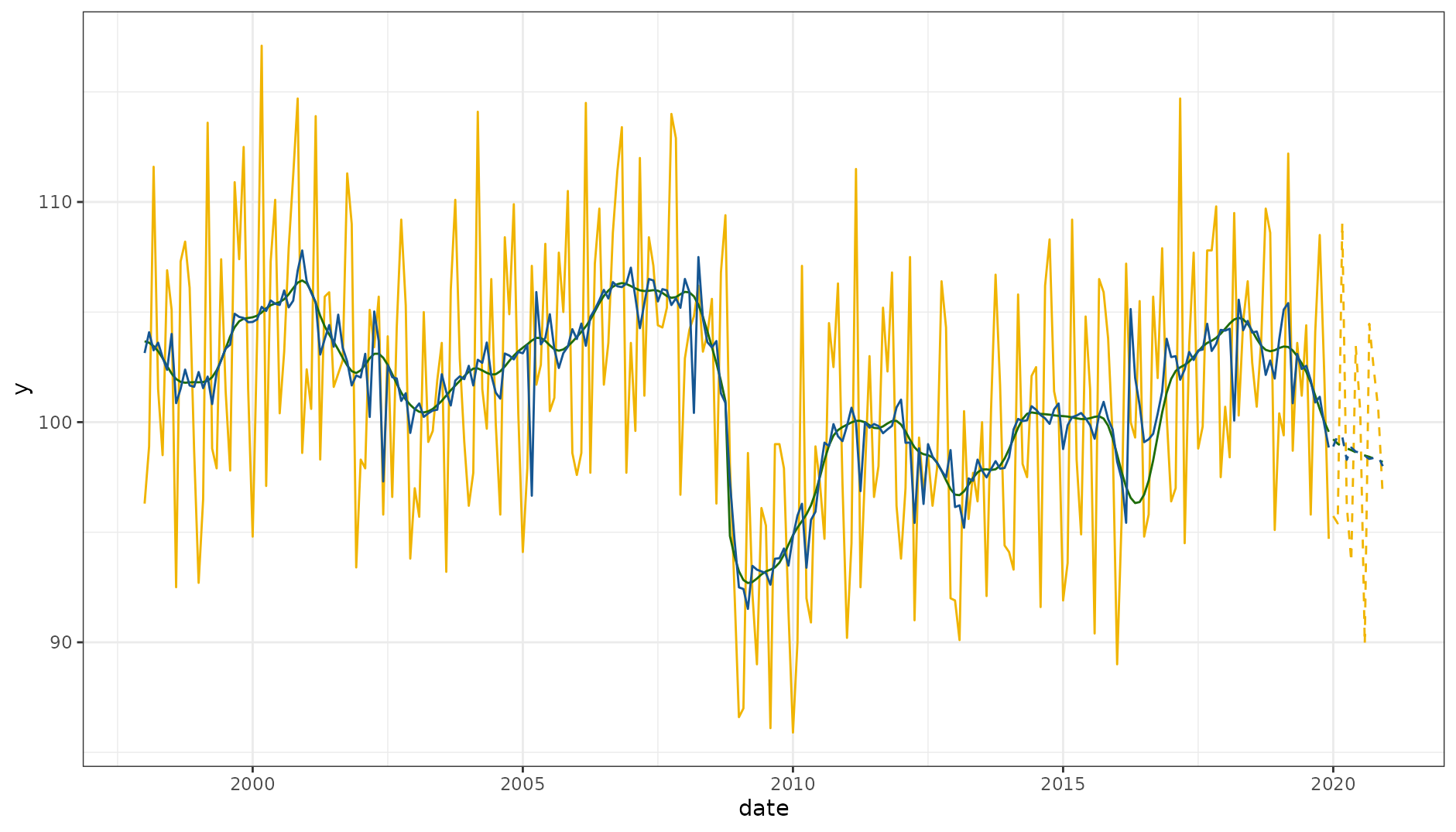SI-ratio plots can be plotted with siratioplot and ggsiratioplot:

ggsiratioplot(mod)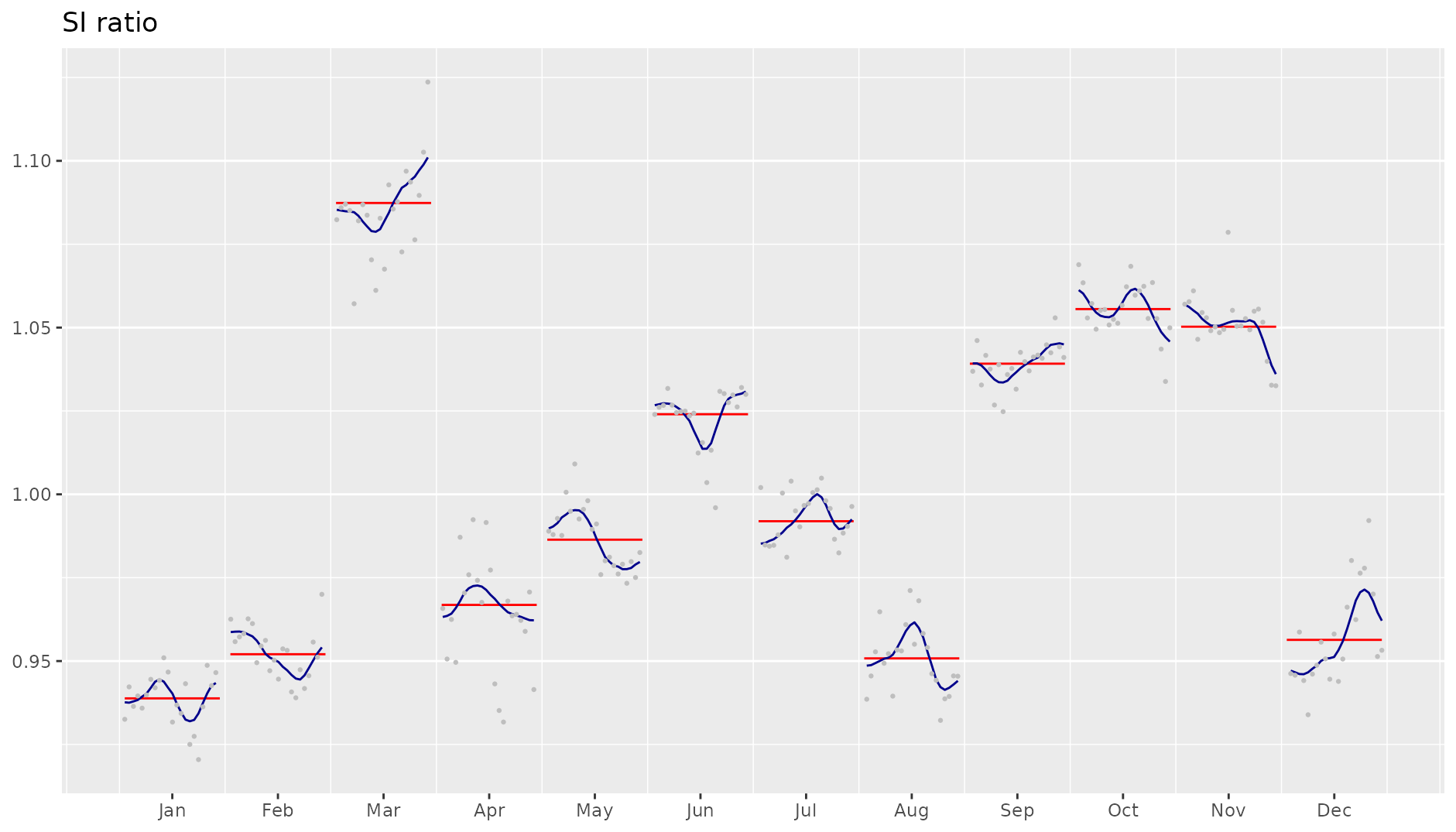And there is also an autoplot() function:

autoplot(mod)